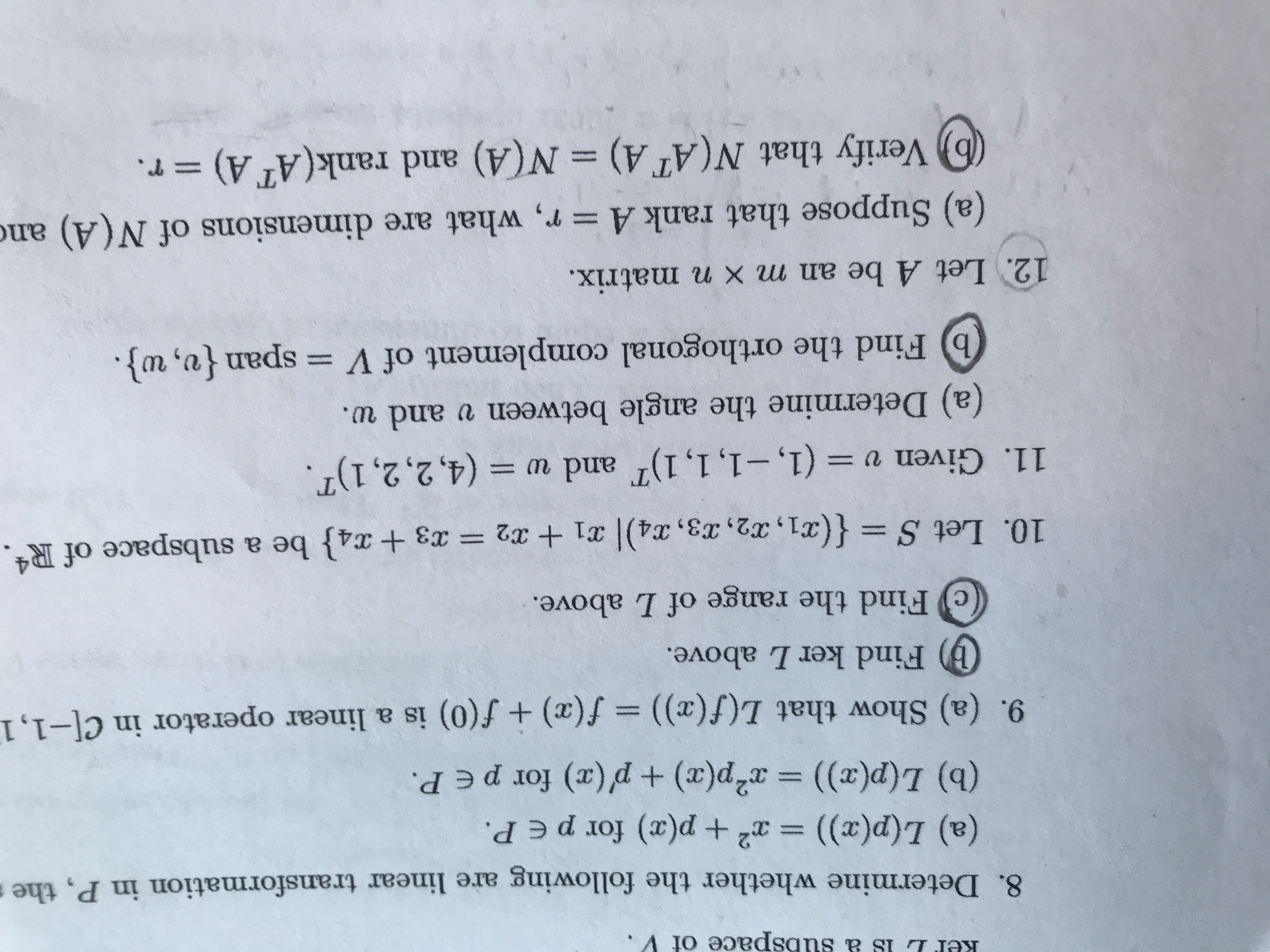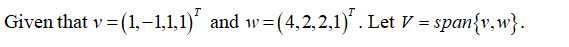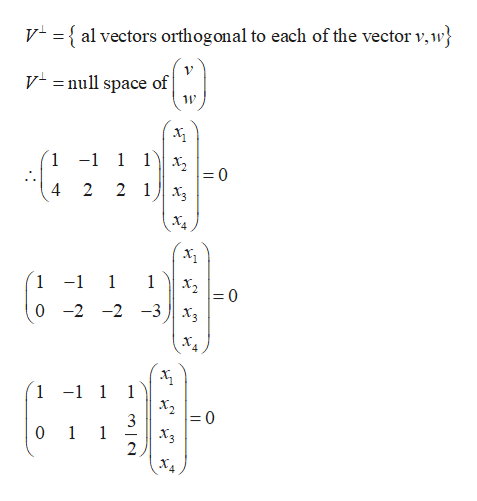# ubspace of V8. Determine whether the following are linear transformation in P, the(a) L(p(x)) = x +p(x) for p e P.(b) L(p(x)) x2p(x) p(x) for peP.9. (a) Show that L(f(x)) = f(x) + f(0) is a linear operator in C[-1,1(b) Find kerL above.Find the range of L above.10. Let S {(x1, 2, 3 , X4)| 31 + x2 = 3 + x4} be a subspace of R4.11. Given v (1,-1, 1, 1) and w (4,2,2,1).(a) Determine the angle between v and w.b Find the orthogonal complement of V = span {v, w}.12. Let A be an m x n matrix.(a) Suppose that rank A = r, what are dimensions of N(A) andb Verify that N(AT A)N(A) and rank(AT A)r.

Question
18 views

11bhelp_outlineImage Transcriptioncloseubspace of V 8. Determine whether the following are linear transformation in P, the (a) L(p(x)) = x +p(x) for p e P. (b) L(p(x)) x2p(x) p(x) for peP. 9. (a) Show that L(f(x)) = f(x) + f(0) is a linear operator in C[-1,1 (b) Find kerL above. Find the range of L above. 10. Let S {(x1, 2, 3 , X4)| 31 + x2 = 3 + x4} be a subspace of R4. 11. Given v (1,-1, 1, 1) and w (4,2,2,1). (a) Determine the angle between v and w. b Find the orthogonal complement of V = span {v, w}. 12. Let A be an m x n matrix. (a) Suppose that rank A = r, what are dimensions of N(A) and b Verify that N(AT A) N(A) and rank(AT A) r. fullscreen
check_circle

star
star
star
star
star
1 Rating
Step 1Step 2

The orthogonal comple...help_outlineImage TranscriptioncloseV = { al vectors orthogonal to each of the vector v,w} Vnull space of 11 1 -1 1 1 х, - 0 4 2 2 1x 1 -1 1 1 х, - 0 0-2 -2 -3x, 1 -1 1 1 х, 0 1 1 Xз ХА fullscreen

### Want to see the full answer?

See Solution

#### Want to see this answer and more?

Solutions are written by subject experts who are available 24/7. Questions are typically answered within 1 hour.*

See Solution
*Response times may vary by subject and question.
Tagged in

### Math